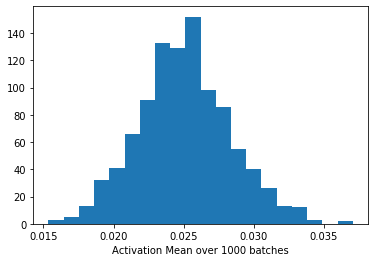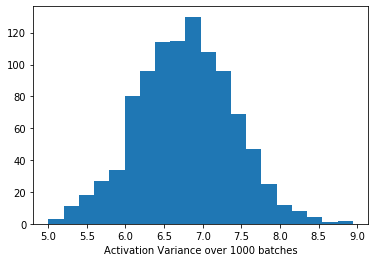# Trained ResNet doesn't work in eval mode, behaves strangely

I’ve trained the stock torchvision ResNet50 to predict classes for images. It achieves 93% accuracy in training.

When I load my model after training and place it in eval mode it gives completely different results, and so accuracy for the same images. The model in .train() mode gives the expected ~93% but in .eval() mode it gives only 50%, as if it hasn’t been trained at all.

I can force the two models (in train and in eval modes) to behave similarly if I run:

``````for module in model.modules():
if isinstance(module, torch.nn.modules.BatchNorm2d):
module.running_mean = None
module.running_var = None
``````

where `model` is the model in eval mode.

I now get the correct outputs from the .eval() model but only if I use the same batch size as in training (64). The output should not depend on batch size.

Is this an issue with the BatchNorm implementation, or am I doing something wrong?

Based on your description it seems that the running stats in batchnorm layers are not able to represent the mean and std of the activations for the validation dataset.
This could happen, if the training and validation datasets do not have similar statistics, which could happen by e.g. a bad dataset split, datasets sampled from different domains, missing preprocessing in the validation dataset etc.

You could try to change the `momentum` of the batchnorm layers and see, if the stats would represent the activations better. If you remove the internal stats I guess that the batch stats would be used, so it’s expected that the results would thus depend on the batch size.

Thanks for the reply! I’m actually having this problem even when using the exact same data, no train/test split.

For example, I initialise two copies of the model and load the trained weights into both - in the first I do `model1.train()`, in the other I do `model2.eval()`. I then load a single batch of data and pass to both models. `model1` will achieve the correct 93% accuracy in this case, as it did in training, as long as the batch size is the same as in training (64). `model2` will achieve 50% regardless of batch size.

Thanks for the update. This artifact might then be caused by different distributions even in the training data. It might be similar to this behavior, i.e. the batchnorm stats would converge to the mean of all samples, while your dataset samples might be coming from distributions with different means.
During the training the activations would be normalized using the batch stats, while the running stats would thus be off.

I’ve plotted the activation mean and variance for the very first layer over 1000 batches. The output of this layer is passed to the first BatchNorm layer so these are the means and variances the BatchNorm layer is using. Is this distribution problematic? It seems they follow a sensible distribution.And the variance here (only one media file per post, sorry)Hello,
did anybody found a solution for this problem ?
This looks like the same issue I just posted here Model (resnet34) performs better without model.eval()

I am using the same Data Loader and pre-processing functions and I can achieve very high accuracy in model.train and very low with model.eval.

I am running with Pytorch 1.13.0+cu117

I assume you’ve found a solution already, but to update the forum the problem lay in BatchNorm2d.
I assumed it was behaving differently in train mode (and it was).

the argument ‘track_running_stats’ I assumed was using data tracked only when training, and was therefore causing my model to behave differently in eval.

Fix:

``````self.bn = torch.nn.BatchNorm2d(input_features,track_running_stats=False)
``````

and will behave the same in eval and training.

If you guarantee to use the same batch size. Without running stats the input batch stats will be used to normalize the activation, which then depends on the batch size and is often not desired during deployment (e.g. as often single samples are used).

@ptrblck Hi, I got the same issue using batchnorm2d in ResNet. If I did not set track_running_stats=False, it seems the model get back to random weights after model.eval(). Can I ask what cause this issue? Thank you.

I doubt the model changes its weights in `eval` mode and you can easily check if e.g. by printing the `.abs().sum()` of each weight. You are most likely running into an issue where the running stats cannot converge to the activation stats.